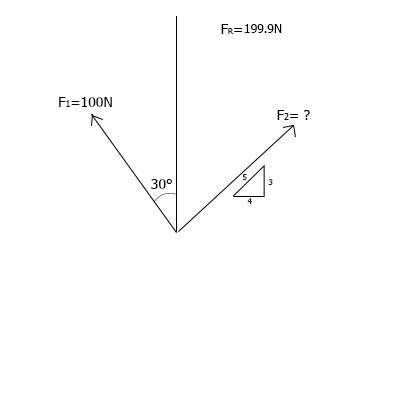# What is the magnitude of force vector?

1. Determine the magnitude of F22. How can I find this one force if I know resultant force and other force? Never done this before, that's why I've no idea.

3. Sorry, I have none.

#### Attachments

Last edited:

$F_r = F_1 + F_2$

The resultant force is the sum of all forces.

I know that, but it's a bit more complicated due to the fact that for these forces I need to find x and y components first.

SteamKing
Staff Emeritus
Homework Helper
Well, what's stopping you from finding the components?

Unknown F2 value.

I came down to:

199.9 = √(0.6x+86.6)2 + (0.8x + 50)2

Though, I can't (don't know how) solve this equations.

NB! I made a mistake and resultant force is 199.9 N.

SteamKing
Staff Emeritus
Homework Helper
Fr = 58.264 N
I don't know where you got 199.9 N

And you could stand an algebra refresher.

Fr = 58.264 N
I don't know where you got 199.9 N

And you could stand an algebra refresher.

Why do you think it's 58.264N? I made a mistake writing it down in the first post.

This is only part of exercise.

There was resultant moment given of 115 N*m, and distance between Fr and point O (where this moment happens) was 0.575 meters. So I divided 115 by 0.575m and I got resultant force of 199.9N.

Now I need to find F2.

Anyone can help with that, please?

SteamKing
Staff Emeritus
Homework Helper
I'm sorry. The OP had a diagram asking to determine the magnitude of F2. If you want to post the correct problem you are working on, please do so.

Now the diagram is correct. Still can't figure out magnitude of F2.

Now the diagram is correct. Still can't figure out magnitude of F2.

It seems to me that you're not even trying. Show us what you've done to find ##F_2##, show us your work.

Last edited:
Chestermiller
Mentor
Now the diagram is correct. Still can't figure out magnitude of F2.
If you call f2 the magnitude of F2, what are its components in the x and y directions (in terms of f2)?

Chet

D H
Staff Emeritus